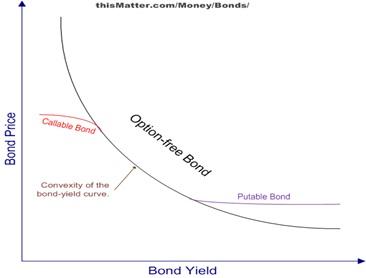# Difference between negative convexity and convexity

I understand that: A bond with less convexity is more affected by interest rates than a bond with more convexity. A bond with less negative convexity will have greater price appreciation when rates decline.

But what is the difference between negative convexity and convexity?

Negative convexityConvexityOk. Positive convexity is when the curve is shape like half moon like the is “(”. duration is just straight line. So when rates goes up prices goes down less when using convexity than duration. when rate goes down bond price goes up more using convexity than duration. The negative convexity shape is like Bear spread lol . Google bear spread shape. When rate goes up bond prices decrease less in convexity than duration just like positive convexitym but when rates goes down the prices of the bond goes up using during but unfortunately it will not goes up at all using convexity because the bond will be call back and your money invested will be on your lap Wondering what to do with them with IR being down. Good ?

And Frank your explination is fair enoughthe exam need shortcut answer

Negative convexity is in the callable region of a callable bond

Bonds normally have negative convexity?

Where the hell is tozerrt when we need him?

avoiding vicky_cool

Here’s my attempt, hopefully someone else can add some additional context to clarify.

Negative Convexity: Price appreciation when rates fall for callable bonds is less than the price appreciation for otherwise equal bonds. The difference in the absolute price between a callable and a noncallble is the callable option value to the bond issuer. As rate continue to fall the option value increases and the chance for the bond to be called is increased. (lower) Negative convexity simply means the price change is less sensitive than what its duration otherwise indicates should occur when interest rates change.

Callable bonds display convexity similar to noncall bonds when rates are rising as the call is out of the money.

Positive Convexity: Price depreciation when rates rise for putable bonds is less than the price depreciation for otherwise equal bonds. The difference in the absolute price between a putable and a nonputable bond is the putable bond option value to the put holder (investor). As rate continue to increase, the value of the putable option increases the and the chance for the bond to be put back to the issuer is increased. (higher) Positive convexity simply means the price movement is more senstive to interest rate changes than what its duration indicates should occur when interest rates decrease and more sensitive to interest rate changes when interest rates increase

Putable bonds display convexity similar to non putable bonds when rates are decreasing as the put is out of the money.

Galli, check this thread i had earlier: http://www.analystforum.com/comment/91601982#comment-91601982 I asked s2000 “if a callable bond has LESS negative convexity (as opposed to a bond with MORE negative convexity) does that mean that when rates decline, price appreciation is more?” His answer was “Yes”. This seems to contradict what you have written above that says: (lower) Negative convexity simply means the price change is less sensitive than what its duration otherwise indicates should occur when interest rates change.

Nyet.

You have the machinery to check this yourself.

Bond A has a price of \$1,010, an effective duration of 5.0 years, and an effective convexity of −50.0 years². What’s its price when its YTM decreases 50bp? What’s its price when its YTM increases 50 bp?

Bond B has a price of \$1,010, an effective duration of 5.0 years, and an effective convexity of −20.0 years². What’s its price when its YTM decreases 50bp? What’s its price when its YTM increases 50 bp?

Sorry what is the answer for those calcs?

Sorry, but those are trivial Level I calculations; you should be able to do those in your sleep.

And that’s the point: rather than asking what happens in this situation, you can work it out yourself. And you’ll remember it ten times as vividly as you would if someone else types in the answers.

-2.625 vs -2.55 (didnt cross check though)

I believe the confusion is on the perspective of what is “more negative” convexity.

Okay thanks s2000 and audacious. I guess i just remember that less negative convexity (as opposed to more negative convexity) means that when rates decline, price appreciation is more. Thank you for clearing up these questions.

I meant, lower negative convexity to mean a more negative number (-50 -> -100)

take a look at this graph, that red line for the callable bond is displaying negative convexity. As rates continue to fall, the comparable change in price is growing less and less sensitive to the change in interest rates in compraision to a option-free bond (that doesn’t display negative convexity). Price is increasing at a decreasing rate in relation to interest rates